﻿ Lifetime Analysis of Titanium Neutral and Singly-Ionized Species in LIBS’s Plasmas: A Physical Chemistry ExperimentPublications are Open
Access in this journal
Article Versions
Export Article
• Normal Style
• MLA Style
• APA Style
• Chicago Style
Original Article
Open Access Peer-reviewed

### Lifetime Analysis of Titanium Neutral and Singly-Ionized Species in LIBS’s Plasmas: A Physical Chemistry Experiment

Alexander Roche, David Shoup, Rosemarie C. Chinni
World Journal of Chemical Education. 2017, 5(6), 197-202. DOI: 10.12691/wjce-5-6-2
Published online: November 27, 2017

### Abstract

In this physical chemistry laboratory, the students develop experience in using Laser-Induced Breakdown Spectroscopy (LIBS) to analyze spectral emission data from to determine lifetimes of neutral and singly-ionized titanium species in LIBS plasmas. LIBS is a spectroscopic technique that focuses a high power laser on a surface. The laser heats, ablates, atomizes, and ionizes the surface material which creates a plasma. The light from the plasma is then spectrally resolved and detected. For LIBS experiments, the data can be collected using gated (using time delays and gate widths) or non-gated detection; this experiment uses gated detection taken at various time delays. The time delays used ranged from 0 to 60 μs to create the time decay curves. After creating the time decay curves for the titanium species used in this experiment, the students were able to examine the curves which illustrated the behavior of the neutral and singly-ionized titanium species and also gained experience calculating lifetimes.

### 1. Introduction

In laser-induced breakdown spectroscopy (LIBS), a high power laser is focused onto a surface. The focused laser pulse heats, ablates, ionizes, and atomizes the surface material and causes the formation of a plasma. The plasma emits light, which is spectrally resolved and detected. The subsequent spectrum can provide both quantitative and qualitative information regarding the sample. LIBS has many advantages including multi-element detection, little or no sample preparation, analysis of solids, liquids, and gases, real time analyses, and a requirement of only optical access to the surface. 1, 2 There are also many applications of LIBS, some of which include metal sorting, heavy metal determination, fissile material detection, geochemical fingerprinting, space exploration, and explosive detection 3, 4, 5, 6, 7, 8.

As the laser is focused onto the sample, its energy couples into the sample, leading to its evaporation. The plasma is formed within the sample’s vapor and the surrounding gas atmosphere which causes excitation of the species in the sample and leads to their spontaneous emission. This further produces a continuum of light which is seen in the first few nanoseconds of plasma formation and causes rising backgrounds LIBS spectra. This continuum decays faster than the spectral emission lines in the plasma. Thus, by delaying the signal collection, the continuum background can be rejected; this is called the time delay, td. 9 The species primarily atomized or ionized in the plasma tend to exist in their neutral or singly-ionized forms, whereas doubly- or triply-ionized species are not likely to be observed in air due to their higher ionization potentials. Neutral or singly-ionized lines are signified by (I) or (II), respectively. Neutral species tend to have a longer lifetime in the plasma over singly-ionized species due to electron-ion recombination, which causes neutral species to remain longer in the plasma, and the decrease in both electron density and plasma temperature at the longer time delays. 1 This lab experiment is designed for students to observe the behavior of neutral and singly-ionized atomic species in the LIBS plasma.

Two LIBS laboratory experiments have been published previously in the Journal of Chemical Education and are in current use at Alvernia. 10, 11 One was designed for an Analytical/Instrumental Analysis course and shows how LIBS can be used to obtain quantitative data through the use of calibration curves. 10 The other was designed for a Physical Chemistry lab where students learned how to calculate plasma temperature and electron density using the spectral information. 11 This lab is also designed for a Physical Chemistry laboratory experiment. It shows the students how singly-ionized and neutral species behave in the plasma and allows them to calculate pseudo-lifetimes of the species for comparative purposes. The actual lab handout supplied to the students is included in the Supplemental Materials Section under Directions for Students. The learning objectives for this experiment are (1) to gain experience using LIBS and work up spectral data, (2) to visually see the difference between singly-ionized and neutral species’ behavior in the plasma, and (3) to learn how to calculate lifetimes using time decay curves.

### 2. Materials and Methods

2.1. Materials

There is no sample preparation for this laboratory experiment. A metal titanium electrode (Metal Electrodes, product code: METELECT12, Miniscience Inc., Clifton, NJ) was used as the sample.

2.2. Equipment

The experimental set-up remained constant throughout this lab experiment. A Nd:YAG laser (λ=1064 nm, pulse width 6 ns) (Surelite II, Continuum, Santa Clara, CA) was operated at 100 mJ per pulse and 10 Hz. The laser pulse was focus onto the sample by a 50 mm focal length lens. The spot size on the sample was approximately 0.080 cm; this produced an approximate power density of 2.5 GW/cm2. The light emitted by the plasma was collected with an optical fiber (QP1000-2-UV-VIS, Ocean Optics, Dunedin, FL) and it was then spectrally dispersed and detected with an echelle spectrograph and ICCD (echelle: SE200, Catalina, Tucson, AZ and ICCD: DH-734-18F-03, IStar, Andor Technology, Belfast, Ireland); the approximate resolving power of the echelle/ICCD is 1700. The data was taken at atmospheric pressure using various time delays ranging from 0 to 60 µs and a constant gate width of 500 ns. The time delay is the time the detector waits to view the pulse and the gate width is how long the detector views each pulse. A 3 second exposure time was used and the signal was integrated on the detector chip, and thus 30 pulses were averaged per trial since the laser was operated at 10 Hz. The timing between the laser and detector were controlled using a digital delay generator (BNC Model 575-4C Digital Delay and Pulse Generator, Berkeley Nucleonic Corp., San Rafael, CA). A typical LIBS set up has been shown previously 10.

2.3. Experimental Procedure and Data Work-up

The students took data on titanium metal using time delays of 0.5, 0.75, 1, 5, 10, 15, 20, 30, 40, 50, and 60 µs. Three spectra were recorded at each time delay, corresponding to a total of 33 spectra. The spectra were saved as text files and supplied to the students. The students used Microsoft Excel for their data work up. Emission line data for two titanium species (a singly-ionized specie and a neutral species) were used for comparison, as shown in Table 1. Time decay curves were created by plotting peak height versus time delay. By creating double exponential fits for the curves using Solver in Microsoft Excel, lifetimes were easily calculated. More information on this is supplied in the Results and Discussion Section.

### 3. Results

The students completed this lab in a two week period. In week 1, students took the LIBS data on their titanium sample but then worked on a different lab. In week 2, the students were given the lab time to do their data analysis during the scheduled lab period. All of the data analysis was compiled in Microsoft Excel. It will take each group approximately 30 minutes to take and save all of their data; therefore, if the lab section is large, it might be hard to complete the whole lab one 3 hour lab period.

The students were directed to create a spreadsheet with all of the raw spectral data. Instead of integrating areas under the emission peaks, the peak heights were used to analyze this data because this can be easily accomplished in Microsoft Excel. The students measured the peak height for each of the titanium lines in each of the replicate trials and recorded an average and standard deviation for each time delay. To view the comparison between the two titanium lines chosen, the students normalized their data sets. Time decay curves were created from this data, plotting the average normalized titanium emission height versus the time delay for each line type on the same graph. The students calculated standard deviations for their normalized data points and the error bars represent the normalized standard deviation from the three trials.

Typical time decay curves are shown in Figure 1. To produce a better predication of the lifetime measurements, double exponential fits were used on the data. Double exponential fits were calculated using the Solver Add-in on Microsoft Excel. Directions are supplied on how to add Solver to Microsoft Excel and how to use Solver for the double exponential fits in the Supplemental Materials. The double exponential fit yields an equation in the form of Equation 1.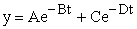(1)

where A and C are considered the amplitudes and B and D are the constants related to the average lifetime. The double exponential fit produces two different lifetimes (τ1 and τ2); τ1 and τ2 are determined by Equation 2 and 3, respectively. Please note: the negative sign is not included with B and D for the lifetime calculation; the negative sign symbolizes that the signal is decreasing as the time delay gets longer, which creates the time decay curve.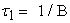(2)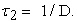(3)

The average lifetime (< τ >) can be calculated using Equation 4. 12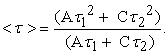(4)

The double exponential fits were verified in MATLAB. The slopes, the individual lifetimes, the R2 values, and the overall average lifetimes are shown in Table 2.

It should be noted that the double exponential fit is not necessary for the singly-ionized titanium line data since a single non-linear exponential fit would produce the same lifetime results for the singly-ionized line data. However, the single non-linear exponential fit does not accurately predict the mid to tail end of the time decay curve for the neutral line, which ultimately skews its lifetime, and therefore, it is necessary to perform a double exponential fit on the neutral line data for a better predicted lifetime prediction. The double exponential fit matches the mid to tail end of the time decay curve for the neutral line and produces a better prediction of results. Ionized lines are excited during the initial formation of the plasma, when the plasma temperature and electron density are the highest, and thus decay quickly and exponentially as the plasma cools, which decreases the plasma’s temperature and electron density. The double lifetime for neutral lines is due to (1) the initial plasma formation which excites both neutral and ionized lines and (2) the electron-ion recombination which causes the neutral lines to remain longer in the plasma. 1, 13 To create less confusion for the students, both sets of data were modeled using the double exponential fits.

View option
• Figure 1. Typical normalized time decay curves for the titanium emissions lines in a LIBS plasma

### 4. Conclusion

This experiment shows the basic behavior difference between neutral and ionized atomic lines in the LIBS plasma. As expected, the students could visually determine that the ionized titanium line decayed quicker than the neutral titanium line by plotting the normalized data on the same graph. The students were also able to discuss the trends in the time decay curves which show as the time delay was increased and the emission peaks subsequently decreased. At the conclusion of the experiment after the results were submitted, the instructor explained why the neutral lines last longer in the plasma.

In summary, the students gained a valuable experience using LIBS and learned about the differences between neutral and ionized lines and their behavior in a LIBS plasma. They were taught how to compile the data, learned how to normalize data sets as well as to calculate a standard deviation for the normalized average values, and learned how to use Solver to perform the double exponential fits, which enabled them to calculate lifetimes for the species. These results are relevant for any physical chemistry course. Moreover, this experiment presents an additional way to incorporate LIBS into the undergraduate laboratory. This laboratory experiment met all of its learning objectives.

### Acknowledgements

This work was funded through U.S. Department of Energy, Office of Science, and Alvernia University. The authors would also like to thank Arthur Halpern for his advice on single exponential linear and single & double nonlinear exponential fits.

### References

  Cremers, D.A. and Radziemski, L.J. Handbook of Laser-Induced Breakdown Spectroscopy, John Wiley & Sons, Ltd, West Sussex, England, 2006. In article View Article  Musazzi, S. and Perini, U. Laser-Induced Breakdown Spectroscopy, Theory and Applications, Springer Series in Optical Sciences, Springer, Verlag Berlin Heidelberg, 2014. In article View Article  Noll, R., Bette, H., Brysch, A., Kraushaar, M., Mönch, I., Peter, L., and Sturm V. “Laser-Induced Breakdown Spectrometry - Applications for Production Control and Quality Assurance in the Steel Industry.” Spectrochim. Acta Part B, 56 (6). 637-649. 2001. In article View Article  Senesi, G.S., Dell’Aglio, M., Gaudiuso, R., De Giacomo, A., Zaccone, C., De Pascale, O., Miano,T.M., and Capitelli M. “Heavy metal concentrations in soils as determined by laser-induced breakdown spectroscopy (LIBS), with special emphasis on chromium.” Environ. Research, 109 (4). 413-420. 2009. In article View Article  PubMed  Cremers, D.A., Beddingfield, A., Smithwick, R., Chinni, R.C., Jones, C.R., Beardsley, B., and Karch L. “Monitoring Uranium, Hydrogen, and Lithium and Their Isotopes Using a Compact LIBS Probe and High Resolution Spectrometer” Appl. Spec. 66 (3). 250-261. 2012. In article View Article  PubMed  Harmon, R.S., Remus, J., McMillan, N.J., McManus, C., Collins, L., Gottfried, J.L., DeLucia Jr., F.C., and Miziolek A.W. “LIBS analysis of geomaterials: Geochemical fingerprinting for the rapid analysis and discrimination of minerals.” Appl. Geochem., 24 (6). 1125-1141. 2009. In article View Article  Mezzacappa, A., Melikechi, N., Cousin, A., Wiens, R.C., Lasue, J., Clegg, S.M., Tokar, R.; Bender, S., Lanza, N.L., Maurice, S., Berger, G., Forni, O., Gasnault, O., Dyar, M.D., Boucher, T., Lewin, E., and Fabre C. “Application of distance correction to ChemCam laser-induced breakdown spectroscopy measurements.” Spectrochim. Acta Part B, 120. 19-29. 2016. In article View Article  DeLucia Jr., F.C. and Gottfried J.L. “Classification of explosive residues on organic substrates using laser induced breakdown spectroscopy.” Appl. Opt., 51 (7). B83-B92. 2012. In article View Article  PubMed  Noll, R. Laser-Induced Breakdown Spectroscopy, Chapter 2, Springer, Verlag Berlin Heidelberg, 2012. In article View Article  Chinni, R.C. “A Simple LIBS (Laser-Induced Breakdown Spectroscopy) Laboratory Experiment to Introduce Undergraduates to Calibration Functions and Atomic Spectroscopy.” J. Chem. Ed., 89 (5). 678-680. 2012. In article View Article  Najarian, M.L. and Chinni R.C. “Temperature and Electron Density Determination on Laser-Induced Breakdown Spectroscopy (LIBS) Plasmas: A Physical Chemistry Experiment.” J. Chem. Ed., 90 (2). 244-247. 2013. In article View Article  Lakowicz, J.R. Principles of Fluorescence Spectroscopy, Plenum Press, New York, 1983, 82-86. In article View Article  Anabitarte, F., Cobo, A., and Lopez-Higuera, J.M. “Laser-Induced Breakdown Spectroscopy: Fundamentals, Applications, and Challenges.” ISRN Spec. Volume 2012, Article ID 285240, 12 pgs, 2012. In article View Article

### Supporting Information

Directions for Solver and Double Exponential Fit
If solver is not part of Microsoft Excel, you need to add it.

Go to File, select options, select Add-in, in the dialog box select Solver Add-In and then Go.

A dialog box pops up; select “Solver Add in.”

Once added, it will show up under the Data Tab.

To use Solver for Double Exponential Fits for this lab experiment:

You need to create a spreadsheet from your data. Label one column as time delay and the other as your Ti line.

You will need to set up columns labeled coefficient A, coefficient B, coefficient C, and coefficient D.

You will need a column labeled as SS total (sum of squares-SS), SS regression, SS residual, R^2, stdev (residuals).

You will need to provide initial estimates for the coefficients; you can use 1 and 0.5 for A and C, respectively, and -0.5 for both B and D.

Create a column next to the Ti line labeled “A exp(Bx) + C exp(Dx)” and solve the equation using your numbers and the set coefficients. Remember to use a dollar sign before and after the letter to keep the coefficient cells constant in the formula and the time delays are the x-values.

The SS total is equal to the COUNT(y-values) times VARP(y-values). The COUNT function is the total number of data points and the VARP function calculated the variance of the data set.

The SS residuals is equal to SUMXMY2(y-values, fit y-values). This is the sum of squares of the differences between the actual data and the fitted data.

The SS regression is equal to SS total minus SS residuals.

R^2 is equal to the SS regression/SS total.

Stdev(residuals) is equal to the SQRT(SS residuals/COUNT(y-values).

Table S1 shows a sample spread sheet with formulas for further clarification.

Go to the Data Tab in Excel and Select Solver.

Use the following options:

• Set Objective: select the SS residuals cell (in the spreadsheet in Table S1, this would be F6)

• To: Select minimum

• By Changing variable cells: highlight the coefficients A, B, C, and D (in the spreadsheet in Table S2, this would be E2:H2)

• Unselect Box that states “make unconstrained variables non-negative”

• Select a Solving method: use GRG Nonlinear

• You can leave the options as is (you do not need to select them)

Select Solve

Solver Results Box pops up; select keep solver solution and select OK

The best fit data will be shown.

Lab Handout for students: Below is the actual lab handout that the students receive for the lab.

Determining the Lifetime of Titanium Neutral and Singly Ionized Lines in LIBS’s Plasmas

Introduction: LIBS is a spectroscopic technique that focuses a high power laser on a surface. The laser heats, ablates, atomizes, and ionizes the surface material which creates a plasma. The light from the plasma is spectrally resolved and detected. LIBS produces neutral atoms symbolized by (I) and singly ionized atoms symbolized by (II).

The LIBS spectrum can provide a wealth of information in relation to the sample being analyzed. LIBS can be used to qualitatively determine elemental composition of a sample, to quantitatively determine concentrations of unknowns, and to determine temperatures and electron densities of the plasma.

In this experiment, you will analyze neutral and singly ionized titanium lines in order to determine their lifetimes in in the plasma. Titanium emission lines will be analyzed at various time delays to create time decay curves. The time-dependent data points will be graphed as a function of time to produce the time decay curve. The purpose of this lab is to determine if there is difference in the time dependent behavior of neutral and singly ionized and their lifetimes in the plasma.

Experimental: LIBS spectra will be obtained using a titanium metal sample. Six different time delays will be used; they include 500 ns, 750 ns, 1 μs, 5 μs, 10 μs, 15 μs, 20 μs, 30 μs, 40 μs, 50 μs, and 60 μs. The gate width will be kept constant at 500 ns. Three spectra will be taken at each time delay. This will produce a total of 33 spectra. The instructor will go over how to take, save, and export the data.

Data Work-up: Once you get your 33 files, you will need to create a comprehensive spreadsheet of all of the spectral data in order of time delay. Determine the peak height of each titanium emission line listed in Table S2 for each of the spectra. Calculate an average and standard deviation for each time delay for both titanium lines. Normalize the data to the maximum height value among the time delays. When you normalize your data only one point should have a value of 1 and all of the others should be less than one. You can then calculate your standard deviation of your normalized point by using Equation S1. To normalize your data set, you take your individual number and divide by the largest number in the data set. An example is shown in Example 1.

Plot the average normalized heights versus the time delay for each titanium line on the same graph; include error bars for the normalized height. You will need to calculate the standard deviation using the Equation S1 for each of the average normalized height data points; this will give you the variance in the data and be used as the error bars. This applies when dividing z by y (i.e. z/y) and each has their own standard deviation associated with it. An example of calculating the standard deviation is shown in Example 2. Example 3 shows a table of calculated standard deviations from using Example 1’s data set with added values for standard deviation.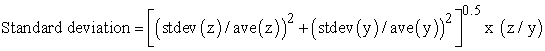(S1)

Plot one graph containing both data sets. Find the lifetimes of the neutral and ionized lines by using double exponential fits. Use the Directions for Solver and Double Exponential Fit handout. An equation is obtained in the form of Equation S2.(S2)

where A and C are considered the slopes and B and D are the constants related to the average lifetime. The double exponential fit produces two different lifetimes (τ1 and τ2); τ1 and τ2 are determined by Equation S3 and 4, respectively. Please note: the negative sign is not included with B and D for the lifetime calculation; the negative sign symbolizes that the signal is decreasing as the time delay gets longer, which creates the time decay curve.(S3)(S4)

The average lifetime (< τ >) can be calculated using Equation S5.(S5)

Example 1:

This is your data set. Normalize the y values.

To normalize the y values, you take the largest y value and divide each y value by it. In Example 1, the largest y value is 1896. To normalize the x=1 row, take 1534 and divide by 1896. To normalize the x=2 row, take 1896 and divide by 1896. To normalize the x=3 row, take 1430 and divide by 1896. To normalize the x=4 row, take 580 and divide by 1896.

Example 2:

Calculate the standard deviation of dividing a by b where a = 1534±158 and b = 1896±205

a/b = 1534/1896 = 0.809

stdev = ((158/1534)2 + (205/1896)2)0.5 x (1534/1896) = 0.121

Example 3:

A complete normalized data set showing the standard deviations for the normalized data.

You would plot the normalized y values on the y-axis and the x-values on the x-axis and then add the standard deviation of your normalized y value for the error bars.

Data Analysis: As part of the data analysis, answer the following questions: What appears to be the relationship between the titanium emission line intensities and time delays? Also, is there a difference between the lifetime of a neutral line and an ionized line? If so, what appears to be the trend? What does this mean?

Published with license by Science and Education Publishing, Copyright © 2017 Alexander Roche, David Shoup and Rosemarie C. ChinniThis work is licensed under a Creative Commons Attribution 4.0 International License. To view a copy of this license, visit http://creativecommons.org/licenses/by/4.0/